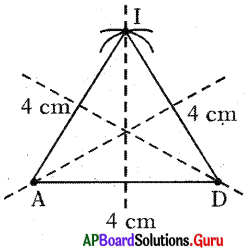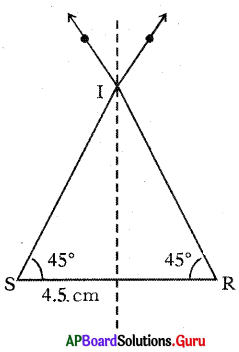SCERT AP 7th Class Maths Solutions Pdf Chapter 12 Symmetry Ex 12.1 Textbook Exercise Questions and Answers.

## AP State Syllabus 7th Class Maths Solutions 12th Lesson Symmetry Ex 12.1

Question 1.
Draw the possible line(s) of symmetry for the following figures, as shown in Figure-(i).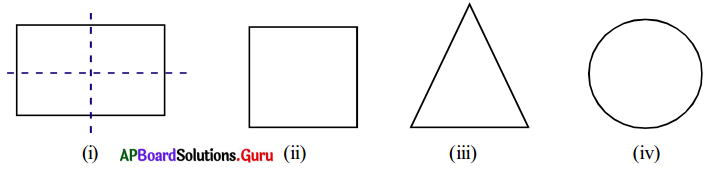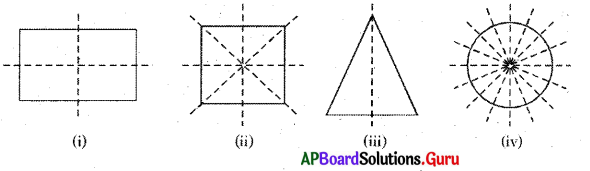Question 2.
Observe the figures with punched holes and draw the axes of symmetry.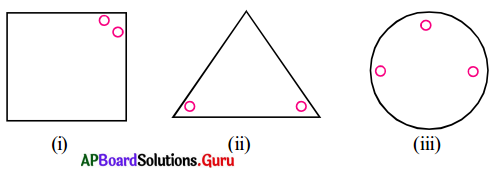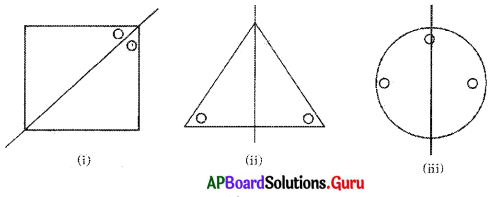Question 3.
Mark the other dot to become the following dotted line in the picture as line symmetry.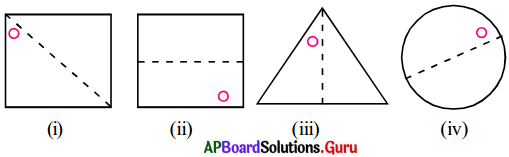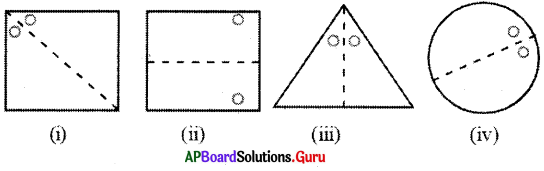Question 4.
State the number of lines ofsyrnrnetry for the following figures and draw them.
(i) An equilateral triangle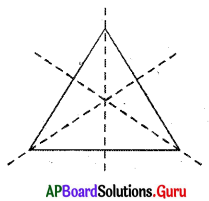Number of lines of symmetry for an equilateral triangle is 3.

(ii) An isosceles triangle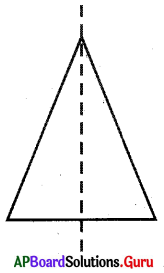Number of lines of symmetry for an isosceles triangle is 1.
(It is the altitude on the unequal side)

(iii) A scalene triangle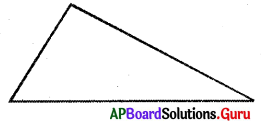We cannot draw the line of symmetry for scalene triangle.

Question 5.
Construct an equilateral triangle with a length of side 4cm and draw all possible lines of symmetry (No need to write the steps of constructions).